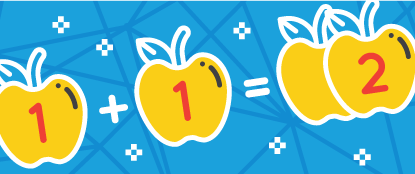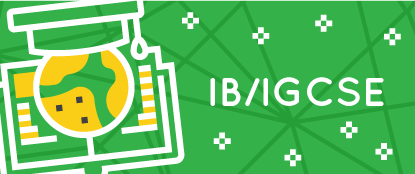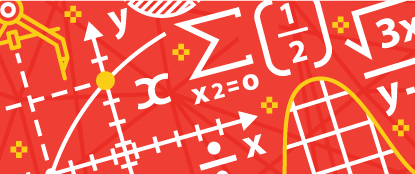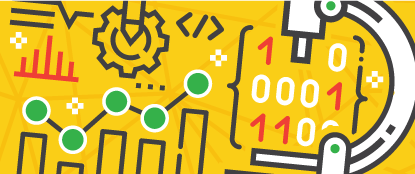# "the awesome ways to learn!"

Math is fun Kickstart your kid’s math journey with us by letting them go through intensive math classes focusing on simple and advanced topics. Students will have individual sessions with our dedicated and qualified trainers and tackle topics they need extra focus on using fun approaches to understand the concepts.

Math everyday Great journey in Discrete Math! We focus on mathematical questions coming from real-life projects that must be solved using logical thinking and computational thinking. Our practices are designed to fit the IB/IGCSE syllabuses which will improve the students’ mathematical and problem-solving skills.

Math for all Our KMath classes are designed to teach students of all ages and mathematical level. It is for those who are just starting to learn math up to those who are joining math competitions. The contents that we offer are up to advanced levels which can support each students’ mathematical requirements they need to accomplish.

Math for life Supported by our top Math trainers, the students will go through different math topics they can relate in real-life. Students will not only memorise formulas and descriptions of mathematical terms, but they will be able to see the beauty of math. Because when we teach students math, we twist it together with technologies and games they love the most!

# “math that is designed for everyone”### Early Age

Making sense of Math for early ages focuses on counting, patterns, problem-solving, estimations, measurements using shapes and other representations.### IB/IGCSE

Go through different levels of Number Theory, Geometry, Functions, Equations, and other Mathematical topics following the IB/IGCSE syllabuses### Applied Math

Learning statistics, probability, optimization, linear algebra, decision trees, and regression. Utilising Python libraries such as SymPy and Sage in Algebra, Analysis, and probability theory.### Data Science

Skills in Data Science and data analysis, data exploration, data computation, and data visualization. Working with tools and libraries such as Numpy, SciPy, SciKit, and Matplotlib.